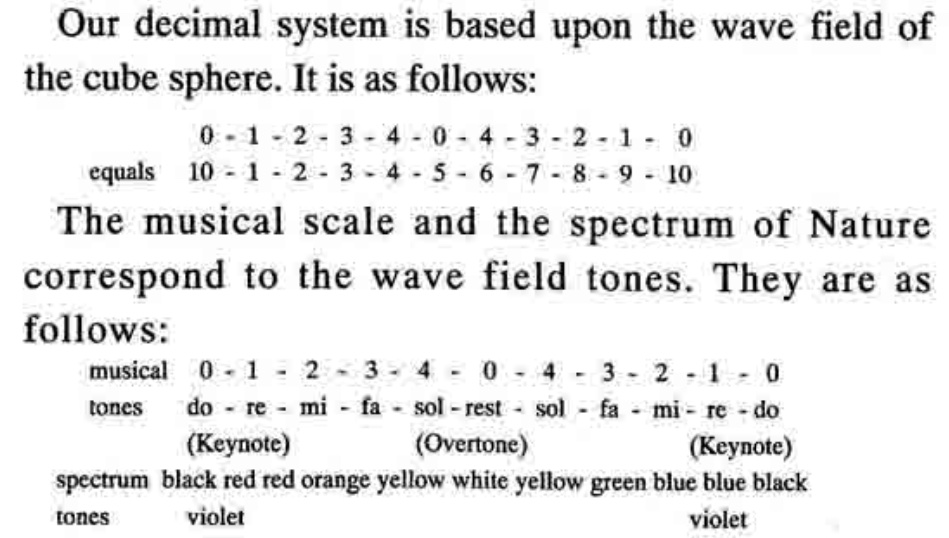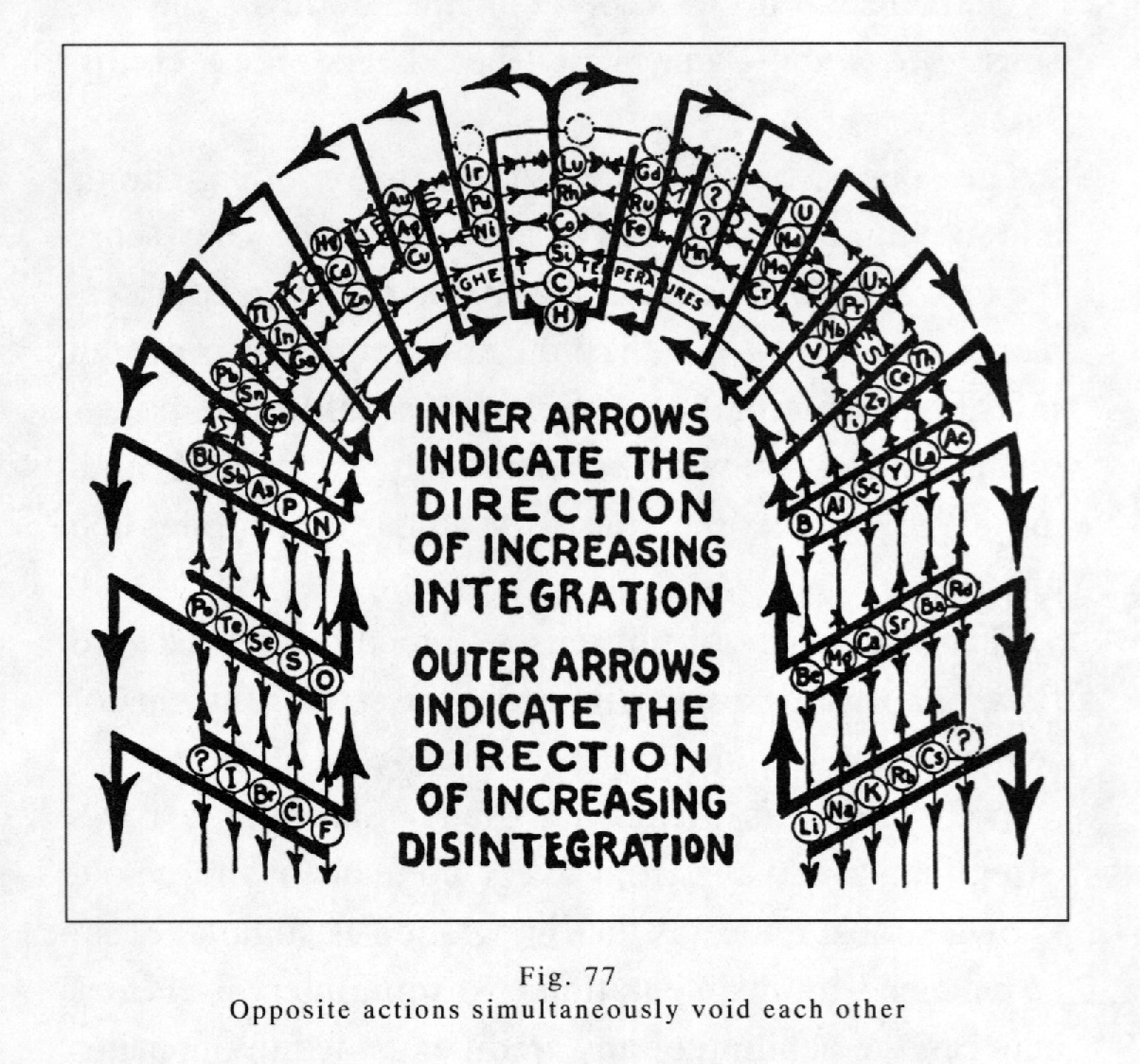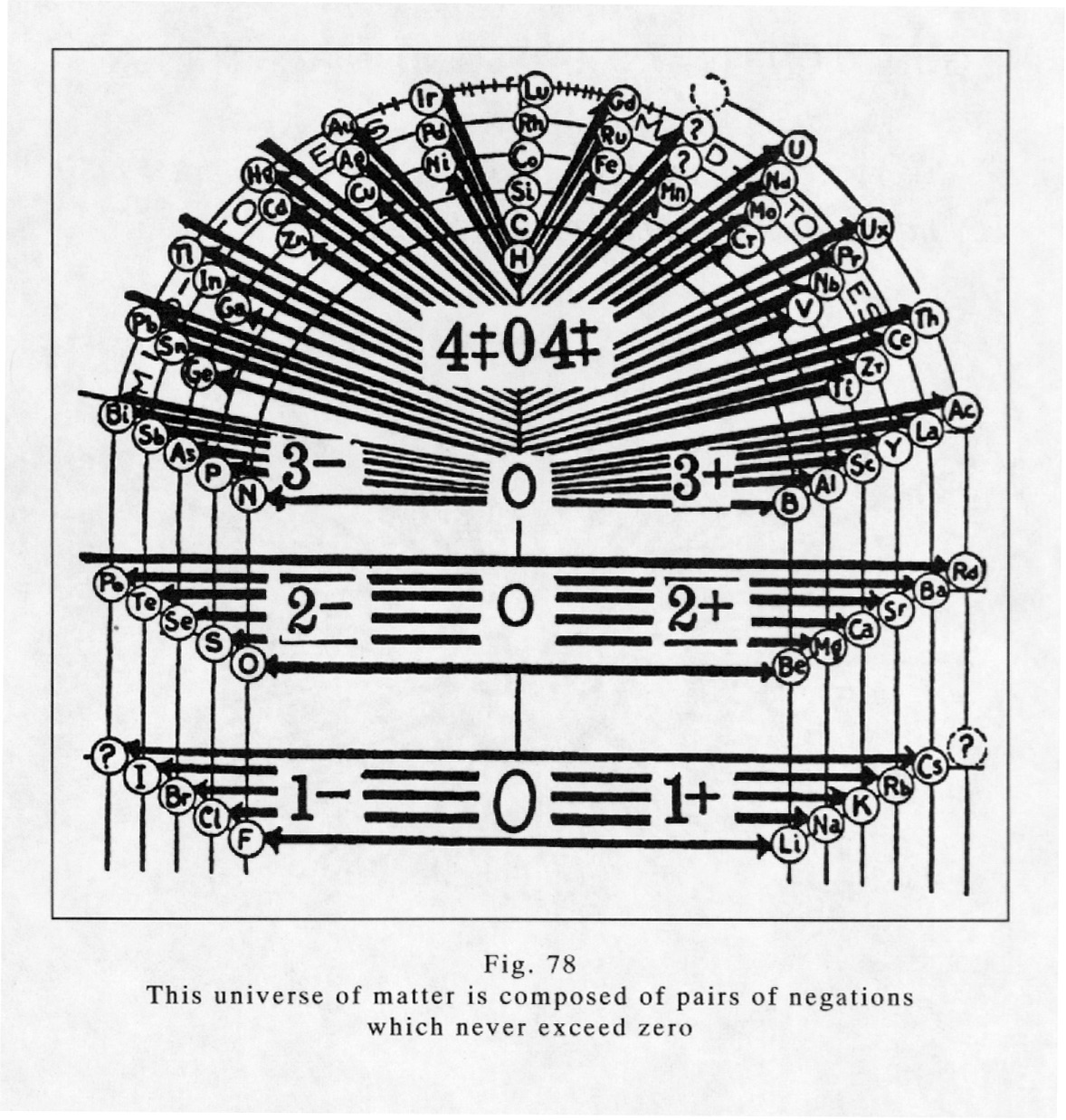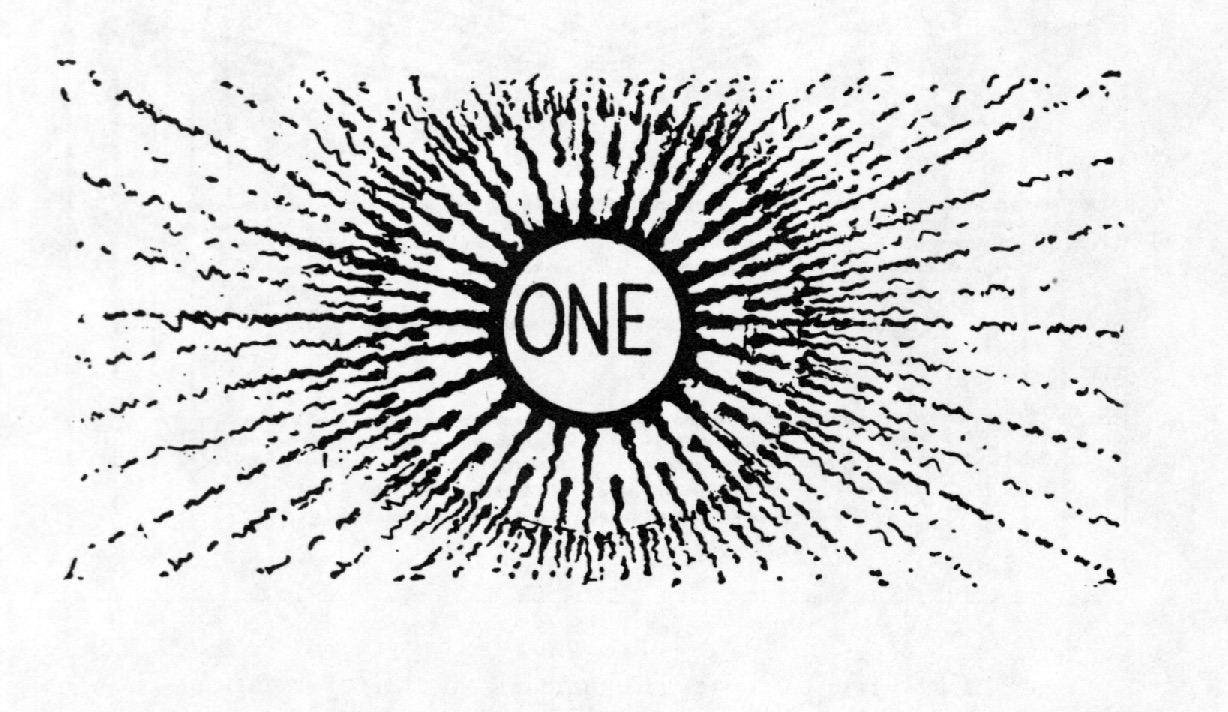# THE VOIDING PRINCIPLE

THE VOIDING PRINCIPLE

This is a zero universe of seeming mechanical motion of force exerted in a seeming three-dimensional universe.

Every action of any nature begins with zero, counts up to nine, to end and begin again at zero. Beyond nine it cannot go, but up to nine it must go. Nine is universal.

Nine is universal because it is the wave field number - the eight of the cube centered by the zero of gravity in the sphere.Fig. 75 demonstrates this fact. The three centering planes are centered by zero. All intersections of these planes add up to eight. Eight, centered by their zero source, equals nine. Likewise the cube itself adds up to eight by counting the intersections of its six faces. Also there are eight directions of action and eight of reaction; each eight being four pairs, which are nine by adding the centering zero.

Nine is the three times three of length, breadth and height extended from zero. The length, breadth and height of any expression is two extended zeros centered by zero. (Fig. 2, p. 219)

Length and breadth are static, for they are both on equipotential levels. Height is dynamic, for it is radial.

The universal nine of matter and space is three mirrors of rest, centered by rest, from which all three extend at right angles to each other, each mirroring itself into the other. Fig. 75

The universal nine of the octave is four pairs of opposite pressures extending diagonally from zero which centers the cube to eight zeros which corner the cube. Fig. 75

The measure of extension from zero to zero is desire for extension. Desire for extension from zero to zero is energy in zero. Energy extended from zero to zero is manifested by pressures of desire equally multiplied and divided - equally added and subtracted - equally credited and debited - and equally and oppositely conditioned. The sum of all these balanced effects is zero. Fig. 75

Zero pressures equally multiplied and divided are manifested by the action and reaction of motion. Motion is a projection of the opposed energy pressures of desire from within a centering zero to the extended mirrors of rest, which measure desire and mirror it back to rest in the centering zero as expressed desire. The sum of dually reflected motion thus expressed is zero.

Zero thus extended by action to fulfill desire for expression, and simultaneously mirrored back to manifest the fulfillment of expressed desire, is all there is to this universe of rest. Zero multiplied or divided - added or subtracted - extended or retracted - results in zero. This is a zero universe in all effects of motion - a seeming universe in time and sequence - and a mirage universe of imagined form.

It is a universe of two negations which simultaneously cancel each other and sequentially repeat the cancelling of their negations to create the illusion that zero can be multiplied - or divided - or added to - or subtracted from - to create a reality which never is nor can become. Fig. 75

That is what Creation is. It is the imagining of knowing. Knowing is Light. Light is still. Imagining is thinking. Thinking is the imagined action and reaction of motion mirrored from zero of rest to zero of rest.

This is a still universe of the Light of knowing. In it is no activity.

But what about our senses? Our senses tell us otherwise. Our senses are inadequate. They deceive us mightily. And that is good, else the play of Creation could not be played. The senses.record but little of the whole. If the senses could but see the whole there would be no play. The senses record motion alone for the senses themselves are but motion. Motion is an illusion which only seems. It has no being.

The senses do not know, but man believes that his senses do know - and in that belief lies man's confusion.

The senses, being but motion, sense moving things and moving light mirrored as moving things. They sense the forward movement of an airplane piling up compression ahead of it - but they do not record the mirrored invisible counterpart of that plane - equal to it in potential and speed - moving backward into a vacuum behind the plane which simultaneously voids the compression ahead of it.

This inadequacy of the senses to record the backward flow of forward moving things - causes the illusions of sequence and of time.In Fig. 77 this principle, diagrammed by arrows extending two ways from every element in the whole known series, indicates that integration is simultaneously balanced by disintegration. No time interval elapses between the debiting of any credit extended to opposites in Nature.Fig. 78 diagrams all matter as pairs of opposite conditions. Each line is connected with its opposite mate. Each of the pair is a negation of the other one. Each pair is conditioned as the two tanks of air in Fig. 18 and the storage battery in Fig. 19, on page 231, are conditioned. Each one of these elemental pairs is an equal and opposite mirrored extension from a centering zero fulcrum.

The fulcrum of all effect is the One Light of God.

GOD ALONE IS - IN MAN - AND IN ALL THINGS.

"Every action is voided as it occurs, is repeated as it is voided, and recorded as it is repeated."
-From THE DIVINE ILIAD [Walter Russell, The Secret of Light, pages 283-288]Created by Dale Pond. Last Modification: Tuesday December 7, 2021 02:48:13 MST by Dale Pond.